Post

# Concept of Sequence in F#

• 11.2k
• 0
• 0

The Concept of Sequence in F# defines that a Sequence is a collection of Elements or Series of Elements. All elements of a Sequence will be of the same type. Sequences are used and useful when you need to operate on a huge amount of data. Sequences are commonly known as Sequence Expressions and are similar to Lists, but when not all elements are used the performance of a Sequence is better than Lists. Both Data structures are used to represent an ordered collection of Elements.

For Lists all elements are computed at once whereas elements of a Sequence are computed when needed. This provides some interesting properties for Sequences such as the ability to represent an Infinite data structure and Lazy evaluation. Sequences are represented by seq<T>type which is an Alias for IEnumerable(T). A seq<type> is simply a value that can be iterated, producing results of type "type" on demand.  Sequences are used to wrap computations, collections and data streams and are frequently used to represent the results of database queries.

# Syntax for Defining a Sequence

The syntax for defining a Sequence is as follows:

seq { expr }

# Example

seq { 0 .. 20 .. 40 .. 100 }

# Sequences using Range Expressions

Range expressions are used to generate a simple Sequence. The default increment for a Range Expression is always 1. The Syntax for that is like below.
seq {n .. m}
If you want to generate a Sequence for Integer ranges with increment of 1. This will take the following form.

# Example

> seq {0..5};;
val it : seq<int> = seq [0; 1; 2; 3; ...]
> seq {0 .. 4}
- ;;
val it : seq<int> = seq [0; 1; 2; 3; ...]
> seq { 0.. 3};;
val it : seq<int> = seq [0; 1; 2; 3]

# Output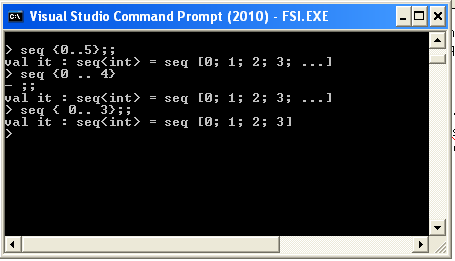F# interactive provides values up to a limit; that is, until 4 values.

# Example

> seq { -2.5 .. 2.5};;
val it : seq<float> = seq [-2.5; -1.5; -0.5; 0.5; ...]

# Output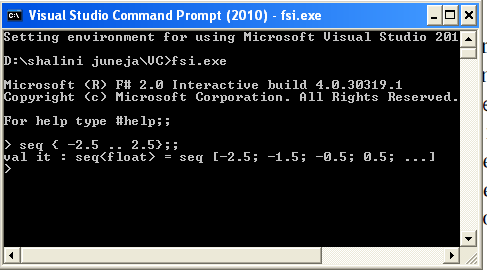An increment of more than one can be used in a Range Expression via "skip" keyword where skip keyword will take the value for an increment.
the syntax is given below.

seq {n .. skip ..m}

# Example

> seq { 1 .. 3.. 8};;
val it : seq<int> = seq [1; 4; 7]

# Output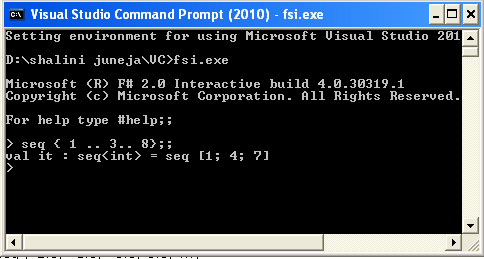# Lazy Evaluation of Sequence

An interesting property of Sequence is lazy evaluation. This property sets Sequence apart from lists. The elements of the Sequence are lazily evaluated, which means in a sequence elements are only evaluated as needed, not all elements at once like Lists. The example showing the difference between the evaluation of elements in list and sequence.

# Example

List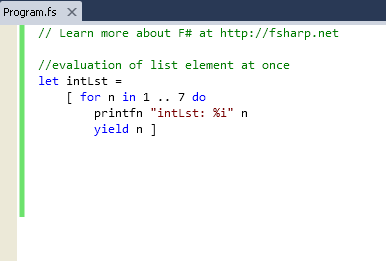//evaluation of list element at once
let intLst =
[ for a in 1 .. 10 do
printfn "intLst: %i" a
yield a ];;

# Output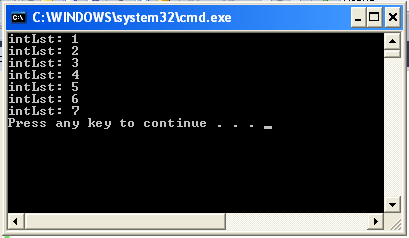# Example

Sequence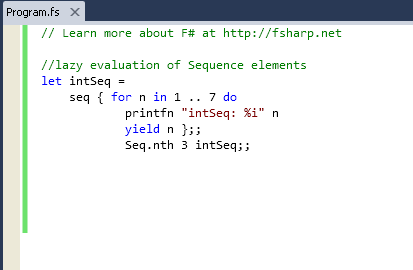//lazy evaluation of Sequence elements
let intSeq =
seq { for n in 1 .. 10 do
printfn "intSeq: %i" n
yield n };;
Seq.nth 3 intSeq;;

# Output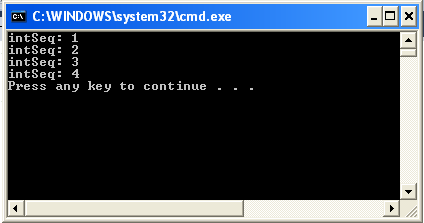# Sequence Comprehensions

An another way of describing a Sequence using a generator function is Sequence comprehensions. Generator functions are of two types simple and complex and both can be used for any kind of container, as the mechanism is the same as the computation expression.

# Syntax

let newSeq = seq {cexpr}

# Iterating a Sequence

If you want to iterate a Sequence you can use for ... in ... do constructs and you can also use the Seq.iter aggregate operator.

# Example

> let range= seq {0 .. 4 ..12};;
val range : seq<int>
> for i in range do
- printfn "i= %d" i;;
i= 0
i= 4
i= 8
i= 12
val it : unit = ()

# Output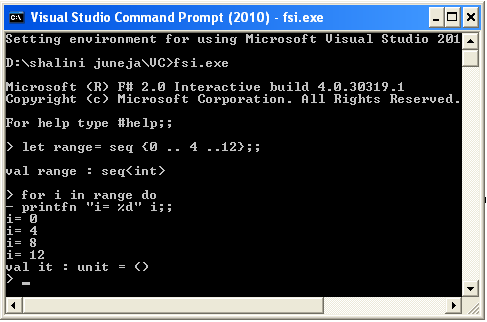# Some important aggregate operators from Seq module

 Aggregate Operator Type Seq.map ('a -> 'b) -> #seq<'a> -> seq<'b> Seq.filter ('a -> bool) -> #seq<'a> -> seq<'a> Seq.iter ('a -> unit) -> #seq<'a> ->unit Seq.empty seq<'a> Seq.singleton 'a -> seq<'a> Seq.truncate int -> #seq<'a> -> seq<'a> Seq.delay (unit -> #seq<'a>) -> seq<'a> Seq.choose ('a -> 'b option) -> #seq<'a> -> seq<'b> Seq.append #seq<'a> -> #seq<'a> -> seq<'a> Seq.concat #seq< #seq<'a> > -> seq<'a> Seq.of_array 'a[] -> seq<'a> Seq.to_array #seq<'a> -> 'a[] Seq.of_list 'a list -> seq<'a> Seq.to_list #seq<'a> -> 'a list

# Converting Sequence

When working with Sequence, it is often good to convert them into a List and Array. If you want to convert a Sequence to an Array or a List you will need to use Seq.to_array and seq.to_list aggregate operator.

# Example

Sequence into Array

let ar= seq { 1..5 } |> //Seq.to_array

# Example

Sequence into List

let list = seq { 1..5 } |> //Seq.to_list